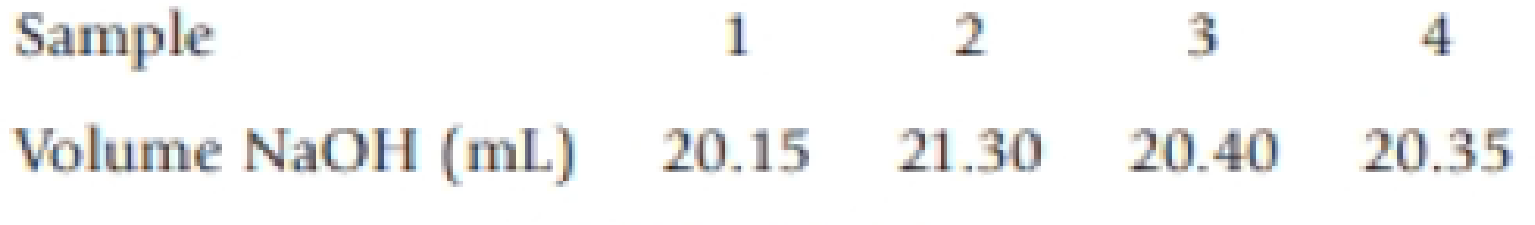Chapter 4, Problem 130IL

Chapter
Section
Textbook Problem

Sulfuric acid is listed in a catalog with a concentration of 95-98%. A bottle of the acid in the stockroom states that 1.00 L has a mass of 1.84 kg. To determine the concentration of sulfuric acid in the stockroom bottle, a student dilutes 5.00 mL to 500. mL She then takes four 10.00-mL samples and titrates each with standardized sodium hydroxide (c = 0.1760 M).(a) What is the average concentration of the diluted sulfuric acid sample? (b) What is the mass percent of H2SO4 in the original bottle of the acid?

a)

Interpretation Introduction

Interpretation:

The average concentration of the diluted sulfuric acid in the sample has to be determined.

Concept introduction:

• The relation between the number of moles and mass of the substance is ,

Numberofmole=MassingramMolarmass

Massingramofthesubstance=Numberofmole×Molarmass

• The molar mass of an element or compound is the mass in grams of 1 mole of that substance, and it is expressed in the unit of grams per mol (g/mol).
• Amountof substance=Concentrationofsubstance×volumeofthesubstance
• Concentrationofthesubstance=AmountofsubstanceVolume
• Mass percent of elements of a compound is the ratio of mass of element to the mass of whole compound and multiplied with hundred.
Explanation

The volume of NaOH required to titrate four 10mL sample of H2SO4 solution is,

Sample 1 2 3 4VolumeofNaOH(mL) 20.15 21.3020.4020

(b)

Interpretation Introduction

Interpretation:

The mass percent of H2SO4 in the original bottle of the acid has to be determined.

Concept introduction:

• The relation between the number of moles and mass of the substance is ,

Numberofmole=MassingramMolarmass

Massingramofthesubstance=Numberofmole×Molarmass

• The molar mass of an element or compound is the mass in grams of 1 mole of that substance, and it is expressed in the unit of grams per mol (g/mol).
• Amountof substance=Concentrationofsubstance×volumeofthesubstance
• Concentrationofthesubstance=AmountofsubstanceVolume
• Mass percent of elements of a compound is the ratio of mass of element to the mass of whole compound and multiplied with hundred.

Still sussing out bartleby?

Check out a sample textbook solution.

See a sample solution

The Solution to Your Study Problems

Bartleby provides explanations to thousands of textbook problems written by our experts, many with advanced degrees!

Get Started